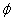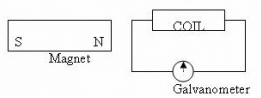NEET  >  Test: Magnetic Flux

# Test: Magnetic Flux

Test Description

## 10 Questions MCQ Test Physics Class 12 | Test: Magnetic Flux

Test: Magnetic Flux for NEET 2022 is part of Physics Class 12 preparation. The Test: Magnetic Flux questions and answers have been prepared according to the NEET exam syllabus.The Test: Magnetic Flux MCQs are made for NEET 2022 Exam. Find important definitions, questions, notes, meanings, examples, exercises, MCQs and online tests for Test: Magnetic Flux below.
Solutions of Test: Magnetic Flux questions in English are available as part of our Physics Class 12 for NEET & Test: Magnetic Flux solutions in Hindi for Physics Class 12 course. Download more important topics, notes, lectures and mock test series for NEET Exam by signing up for free. Attempt Test: Magnetic Flux | 10 questions in 10 minutes | Mock test for NEET preparation | Free important questions MCQ to study Physics Class 12 for NEET Exam | Download free PDF with solutions
 1 Crore+ students have signed up on EduRev. Have you?
Test: Magnetic Flux - Question 1

### A constant current I is maintained in a solenoid. Which of the following quantities will not increase if an iron rod is inserted in the solenoid along its axis?

Detailed Solution for Test: Magnetic Flux - Question 1

When an iron rod is inserted inside a solenoid the magnetic field inside the solenoid increases due to the high magnetic permeability of iron. Due to the increased magnetic field, the magnetic flux also increases. The self-inductance of the solenoid also increases because self-inductance (L) of the coil is directly proportional to the permeability of the material inside the solenoid. The rate of joule heating doesn’t change.

Test: Magnetic Flux - Question 2

### Whenever the magnetic flux () linked with a coil changes the emf is induced in the circuit, This emf lasts

Detailed Solution for Test: Magnetic Flux - Question 2

The induced emf ,E=− dϕ​/dt where ϕ=magnetic flux
Hence, emf will last as long as flux keeps changing.

Test: Magnetic Flux - Question 3

### The expression for the magnetic flux

Detailed Solution for Test: Magnetic Flux - Question 3

Magnetic Flux Formula
Magnetic flux is the product of the average magnetic field times the perpendicular area that it crosses.
Magnetic flux = number of turns x Magnetic field x Area x (angle between the planar area and the magnetic flux)
The equation is:
Φ =N B A cos(θ)
Where:
N=number of turns
Φ: Magnetic Flux
A: Area
B: Magnetic field
θ: angle between a perpendicular vector to the area and the magnetic field

Test: Magnetic Flux - Question 4

A magnet is moved towards the coil (a) quickly and (b) slowly, and then the work done is​

Detailed Solution for Test: Magnetic Flux - Question 4

When a magnet is moved towards the coil quickly, the rate of change of flux is larger than that if the magnetic field is moved slowly, thus larger emf is induced due to quick movement of the coil.

Test: Magnetic Flux - Question 5

A cylindrical bar magnet is held along the axis of a circular conducting loop. Which one of the following movements of the bar magnet will not induce a current in the loop?

Detailed Solution for Test: Magnetic Flux - Question 5

A cylindrical bar magnet is kept along the axis of a circular coil. If the magnet is rotated about its axis, then there is no change in magnetic flux, there is no emf induced in the coil, no current will be induced in the coil.

Test: Magnetic Flux - Question 6

The magnetic flux () linked with a coil is related to the number (N) of turns of the coil as

Test: Magnetic Flux - Question 7

The total number of magnetic lines of force crossing a surface normally is termed as

Detailed Solution for Test: Magnetic Flux - Question 7

The measurement of the total magnetic field that passes through a given area is known as magnetic flux. It is helpful in describing the effects of the magnetic force on something occupying a given area.
If we consider a simple flat area A as our example and angle θ as the angle between the normal to the surface and a magnetic field vector, then the magnetic flux is given by the equation:
ϕ=BAcosΘ

Test: Magnetic Flux - Question 8

In the given circuit the maximum deflection in the galvanometer occurs whenDetailed Solution for Test: Magnetic Flux - Question 8 This question based on lenz law. as the bar magnet is moving , there is a change in magnetic flux.This will give polarity in the coil. Hence the current developed in the coil. so the option d is right.
Test: Magnetic Flux - Question 9

A coil of area 10 cm² of 20 turns is placed in uniform magnetic field of 104 gauss. The normal to the plane of the coil makes an angle of 60° with the magnetic field. The flux in maxwell through the coil is​

Detailed Solution for Test: Magnetic Flux - Question 9

1 gauss=10-4 Tesla
Φ=BANCos θ
Φ=104x104x10x10-4x20xcos60o
=105x20x1/2
=106

Test: Magnetic Flux - Question 10

Find the flux contained by the material when the flux density is 11.7 Tesla and the area is 2 units.

Detailed Solution for Test: Magnetic Flux - Question 10

The total flux is given by φ = ∫ B.ds, where ∫ds is the area. Thus φ = BA. We get φ = 11.7 x 2 = 23.4 units.

## Physics Class 12

157 videos|430 docs|213 tests
 Use Code STAYHOME200 and get INR 200 additional OFF Use Coupon Code
Information about Test: Magnetic Flux Page
In this test you can find the Exam questions for Test: Magnetic Flux solved & explained in the simplest way possible. Besides giving Questions and answers for Test: Magnetic Flux, EduRev gives you an ample number of Online tests for practice

## Physics Class 12

157 videos|430 docs|213 tests

### How to Prepare for NEET

Read our guide to prepare for NEET which is created by Toppers & the best Teachers